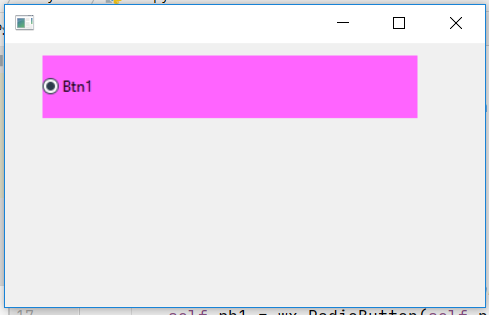Related Articles

# wxPython – Change Size of Radio Button

• Last Updated : 19 May, 2021

In this article we will learn to change size of a Radio Button. We will change the size of Radio Button using SetSize() function associated with wx.RadioButton class of wxPython. SetSize() function is simply used to change the size of the window of Radio Button in pixels.

Parameters:

Code Example:

## Python3

 `import` `wx` `APP_EXIT ``=` `1`  `class` `Example(wx.Frame):` `    ``def` `__init__(``self``, ``*``args, ``*``*``kwargs):``        ``super``(Example, ``self``).__init__(``*``args, ``*``*``kwargs)` `        ``self``.InitUI()` `    ``def` `InitUI(``self``):``        ``self``.pnl ``=` `wx.Panel(``self``)``        ` `        ``# create radio button at position (30, 10)``        ``self``.rb1 ``=` `wx.RadioButton(``self``.pnl, label ``=``'Btn1'``,``                             ``pos ``=``(``30``, ``10``), size ``=``(``100``, ``20``))` `        ``# change background colour``        ``self``.rb1.SetBackgroundColour((``255``, ``100``, ``255``, ``255``))` `        ``# change size to (300, 50)``        ``self``.rb1.SetSize((``300``, ``50``))`  `def` `main():``    ``app ``=` `wx.App()``    ``ex ``=` `Example(``None``)``    ``ex.Show()``    ``app.MainLoop()`  `if` `__name__ ``=``=` `'__main__'``:``    ``main()`

Output Window:Attention geek! Strengthen your foundations with the Python Programming Foundation Course and learn the basics.

To begin with, your interview preparations Enhance your Data Structures concepts with the Python DS Course. And to begin with your Machine Learning Journey, join the Machine Learning – Basic Level Course

My Personal Notes arrow_drop_up